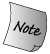### 6.9. do while 语句

We might want to write a program that interactively performs some calculation for its user. As a simple example, we might want to do sums for the user: Our program prompts the user for a pair of numbers and produces their sum. Having generated one sum, we'd like the program to give the user the option to repeat the process and generate another.

The body of this program is pretty easy. We'll need to write a prompt, then read a pair of values and print the sum of the values we read. After we print the sum, we'll ask the user whether to continue.

The hard part is deciding on a control structure. The problem is that we want to execute the loop until the user asks to exit. In particular, we want to do a sum even on the first iteration. The do while loop does exactly what we need. It guarantees that its body is always executed at least once. The syntactic form is as follows:

```     do
statement
while   (condition);
```Unlike a while statement, a do-while statement always ends with a semicolon. 与 while 语句不同。do-while 语句总是以分号结束。

The statement in a do is executed before condition is evaluated. The condition cannot be empty. If condition evaluates as false, then the loop terminates; otherwise, the loop is repeated. Using a do while, we can write our program:

```     // repeatedly ask user for pair of numbers to sum
string rsp; // used in the condition; can't be defined inside the do
do {
cout << "please enter two values: ";
int val1, val2;
cin  >> val1 >> val2;
cout << "The sum of " << val1 << " and " << val2
<< " = " << val1 + val2 << "\n\n"
<< "More? [yes][no] ";
cin  >> rsp;
} while (!rsp.empty() && rsp != 'n');
```

The body of this loop is similar to others we've written and so should be easy to follow. What might be a bit surprising is that we defined rsp before the do rather than defining it inside the loop. Had we defined rsp inside the do, then rsp would go out of scope at the close curly brace before the while. Any variable referenced inside the condition must exist before the do statement itself.

Because the condition is not evaluated until after the statement or block is executed, the do while loop does not allow variable definitions:

```     // error: declaration statement within do condition is not supported
do {
// ...
mumble(foo);
} while (int foo = get_foo()); // error: declaration in do condition
```

If we could define variables in the condition, then any use of the variable would happen before the variable was defined!

## Exercises Section 6.9

 Exercise 6.17: Explain each of the following loops. Correct any problems you detect. 解释下列的循环。更正你发现的问题。 ``` (a) do int v1, v2; cout << "Please enter two numbers to sum:" ; cin >> v1 >> v2; if (cin) cout << "Sum is: " << v1 + v2 << endl; while (cin); (b) do { // ... } while (int ival = get_response()); (c) do { int ival = get_response(); if (ival == some_value()) break; } while (ival); if (!ival) // ... ``` Exercise 6.18: Write a small program that requests two strings from the user and reports which string is lexicographically less than the other (that is, comes before the other alphabetically). Continue to solicit the user until the user requests to quit. Use the string type, the string less-than operator, and a do while loop. 编写一个小程序，由用户输入两个 string 对象，然后报告哪个 string 对象按字母排列次序而言比较小（也就是说，哪个的字典序靠前）。继续要求用户输入，直到用户请求退出为止。请使用 string 类型、string 类型的小于操作符以及 do while 循环实现。